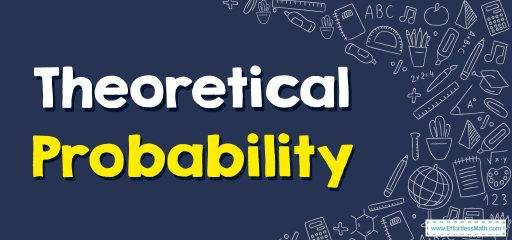# How to Solve Theoretical Probability?

Theoretical probability can be defined as the number of favorable outcomes divided by the total number of possible outcomes. In this guide, you will learn more about the definition of theoretical probability.## A step-by-step guide tosolving theoretical probability

Theoretical probability, as the name suggests, is the theory behind probability. It tells us what should happen in an ideal situation without doing any experiments.

Theoretical probability can be defined as the number of favorable outcomes divided by the total number of possible outcomes. No experiment is needed to determine the theoretical probability.

### How to find theoretical probability?

Theoretical probability is used to express the probability of an event occurring without performing any experiment. Suppose someone has $$20$$ lottery tickets and a total of $$600$$ tickets are sold. The steps to calculate the theoretical probability of winning a prize are as follows:

Step 1: Identify the number of favorable outcomes. Since there are $$20$$ lottery tickets, $$20$$ will be the desired number of results.

Step 2: Determine the total possible outcomes. Since a total of $$600$$ tickets were sold in this way, $$600$$ will be the total number of possible results.

Step 3: Divide the value from step $$1$$ by step $$2$$ to calculate the theoretical probability. Therefore, $$\frac{20}{600}=0.03$$. This indicates that the probability of a person winning a lottery prize is $$0.03$$.

### Theoretical Probability – Example 1:

Find the probability of getting $$2$$ on a fair die.

Solution:

The possible outcomes of rolling a die are $$1, 2, 3, 4, 5, 6$$, so the total number of outcomes $$=6$$. As we want to calculate the probability of getting only $$2$$, therefore, the number of desired outcomes $$= 1$$.

$$p\left(2\right)=\frac{1}{6}=0.16$$

### What people say about "How to Solve Theoretical Probability? - Effortless Math: We Help Students Learn to LOVE Mathematics"?

No one replied yet.

X
30% OFF

Limited time only!

Save Over 30%

SAVE $5 It was$16.99 now it is \$11.99